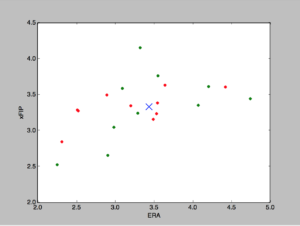## Perceptron Code

I used two different codes to make the perceptron. The first is from this blog post and the second is from some work I did with Stephanie Koulton.

``````import numpy as np

X = np.array([
[-2,4,-1],
[4,1,-1],
[1, 6, -1],
[2, 4, -1],
[6, 2, -1],

])

y = np.array([-1,-1,1,1,1])

def perceptron_sgd(X, Y):
w = np.zeros(len(X))
eta = 1
epochs = 20

for t in range(epochs):
for i, x in enumerate(X):
if (np.dot(X[i], w)*Y[i]) <= 0:
w = w + eta*X[i]*Y[i]

return w

w = perceptron_sgd(X,y)
print(w)``` And here is some of the code I created with Stephanie```

import random
import numpy as np

def guess(sumtotal):
if sumtotal < 0:
return 0
else:
return 0

train_data = np.array([
([0,0],0),
([0,1],1),
([1,0],1),
([1,1],1)
])

biasinput = 1
weight = np.random.rand(2)
biasweight = np.random.rand(1)
const = 0.2

def perceptron():
x, expected = random.choice(train_data)
result = np.dot(x,weight) + np.dot(biasinput, biasweight)
print(result)
error = expected – guess(result)
print(error)
errors.append(error)
newweight = const*error*x
#delta = np.dot(x, error)
#print(delta)
#weight = weight + delta * const
#print(w)

perceptron()

## K-Means Clustering with ERA and xFIP

Coding is not an easy thing for me. Learning Python, P5.js, C#, C++, etc have been no different than learning French, Japanese, or Dutch to me. I don’t say this to invoke pity but rather to explain why frequent examples of mine are about baseball. If I apply baseball sabermetrics (a passion of mine) to coding, the work becomes less ‘work’ and more an interesting study of baseball. This assignment was no different.

While the concepts of K-Means Clustering are something I’m a bit more familiar with thanks to Stephanie’s recording of your class and additional research, the notion of building a proper algorithm without a previously coded framework is really scary and daunting to me. Rather than try to code one myself, I figured it’d be a better usage of time to find previously existing code (to which of course I would site the creator of that code), and figure out interesting ways in which I could use it.

The code featured below was created by a YouTuber whose work is featured here. I chose this example because the author explained each line of code as he created it so I was able to understand what was happening a bit better.

For my data in this example, I decided to take two points from pitchers in baseball: ERA and xFIP. I’m sure you’re familiar with the former – ERA is merely earned run average or the total amount of earned runs given up in a game by a pitcher, divided by the number of innings he pitched and multiplied by nine – but xFIP might be a bit trickier to non-baseball nerds. xFIP is a sabermetric used to better determine the true ability of a pitcher. It is a metric that strips away the defense of the team the pitcher plays on, the amount of “luck” he has, and normalizes the amount of home runs he gives up based off of the league average. For a more in depth explanation, check this page out.

I took the ERA and xFIP from the top 10 American League and National League pitchers and turned them into X,Y data points. I then used the code below to create a K-Means Cluster and got this:So the print function lets me know that the Cluster is located at (3.49, 3.34). My issue is I’m not sure what can be drawn from this information. Does this mean the average pitcher of the top 10 pitchers in both the American and National league has an ERA of 3.49 with an xFIP of 3.34? I’m fairly sure K-Means is different than a mere average but what does the information reveal?

Also, I’d love to figure out how to not just find two clusters (I know how to do that in the code, just change the number in kmeans = KMeans(n_clusters = 1) to 2) but to find the K-Mean cluster for the AL pitcher and NL pitcher individually.

Here is the code that was used for the above:

import numpy as np
import matplotlib.pyplot as plt
from sklearn.cluster import KMeans

_ALpitchers = np.array([
[2.25, 2.52],
[2.90, 2.65],
[2.98, 3.04],
[3.29, 3.24],
[4.07, 3.35],
[4.74, 3.44],
[3.09, 3.58],
[4.20, 3.61],
[3.55, 3.76],
[3.32, 4.15]
])

_NLpitchers = np.array([
[2.31, 2.84],
[3.49, 3.15],
[3.53, 3.23],
[2.52, 3.27],
[2.51, 3.28],
[3.20, 3.34],
[3.54, 3.38],
[2.89, 3.49],
[4.42, 3.60],
[3.64, 3.63]
])

kmeans = KMeans(n_clusters = 1)
kmeans.fit(_ALpitchers, _NLpitchers)
centroids = kmeans.cluster_centers_
labels = kmeans.labels_

print(centroids)
print(labels)

colors = [‘g.’,’g.’,’g.’]
colors_two = [‘r.’, ‘r.’, ‘r.’]

for i in range(len(_ALpitchers)):
print(“coordinate:”, _ALpitchers[i], “label:”, labels[i])
plt.plot(_ALpitchers[i], _ALpitchers[i], colors[labels[i]], markersize = 10)

for g in range(len(_NLpitchers)):
print(“coordinate:”, _NLpitchers[g], “label:”, labels[g])
plt.plot(_NLpitchers[g], _NLpitchers[g], colors_two[labels[g]], markersize = 10)
plt.scatter(centroids[:,0], centroids[:,1], marker = ‘x’, s= 150, zorder = 10)